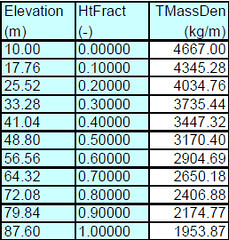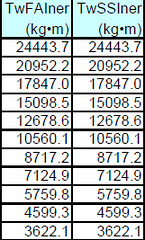# Inertial Moments of OC3-Hywind Components

I have a few questions regarding the inertia moments given for the OC3-Hywind:

• How were these numbers calculated?
• Not all of them were presented (eg. Tower shows no yaw-axis inertia). Was there a reason for this?

I’m looking to determine the radii of gyration of the overall tower system (rotor, nacelle, tower, platform/ballast) about axes originating at the initial position of the tower (tower-centered in X and Y; at the SWL for Z). Does anyone have these radii or the inertial moments of the components about these axes?

Thanks!
Sean

Dear Sean,

Which inertias of the OC3-Hywind system are you referring to when you ask how they were calculated?

The tower yaw inertia can be calculated at each cross section as the sum of the TwFAIner and TwSSIner values from Table 2-1 in the OC3-Hywind specifications report.

Although this data is not in the OC3-Hywind specifications report, I have calculated the aggregate mass/inertia of the total system (platform + ballast + tower + nacelle + rotor)—not including the moorings—using MSC.ADAMS. (I have never computed the aggregate properties including the mooring system.) The output from MSC.ADAMS is given below. You can use these to derive the radii of gyrations that you want. The center of mass location, orientation, and inertias in this output are all relative to the coordinate system described in the OC3-Hywind report. This system originates at the tower centerline at still water level with “X” pointing downwind, “Y” pointing to the left when looking downwind, and “Z” pointing vertically upwards.

The aggregate mass relative to ._127_ADAMS.Ground_P.InertialFrameCS_M is:
Mass : 8.0660481545E+006 kg
Center of Mass :
Location : -1.3918125391E-002, 1.1132995542E-002, -78.001301464 (meter, meter, meter)
Orientation : 231.3122968126, 6.8230961454E-002, 88.6142095906 (deg)
Mass Inertia Tensor :
IXX : 6.8025977471E+010 kg-meter2
IYY : 6.8022535284E+010 kg-meter
2
IZZ : 1.9157300092E+008 kg-meter2
IXY : 9.9135213851E+006 kg-meter
2
IZX : -8.6878157798E+006 kg-meter2
IYZ : 6.965876724E+006 kg-meter
2

These inertias are computed in a vacuum. While damping does not contribute to the inertia, added mass will. Figure 4-4 shows the added mass matrix as a function of the platforms oscillation frequency.

I hope that helps.

Best regards,

Dr. Jonkman,

This is precisely the data I was looking for! Thanks so very much!

Thanks!
Sean

Dr. Jonkman,

I do, actually, have one followup question: What is the meaning of the “Orientation” of the CM?

Thanks!
Sean

Dear Sean,

In the MSC.ADAMS output, the “Orientation” is not referring to the CM, but to the orientation of the principal axes of inertia.

Best regards,

Hi both,

As Jason has pointed out that the tower inertia tensor property has been specified in the OC3-Hywind document, which might be the following columns in Table 2-1.However, I am not quite sure how to use these values. For example, in order to calculate the tower fore-aft moment of inertia about tower bottom, I guess it maybe follows the calculation procedure below.

+(24443.7+20952.2)/27.76+(4667.00+4345.28)/27.763.88^2
+(20952.2+17847.0)/2
7.76+(4345.28+4034.76)/27.76(3.88+7.76)^2
+…
+…
+…
+(4599.3 +3622.1 )/27.76+(2174.77+1953.87)/27.76*(3.88+7.76*9)^2

Is it right?

Besides, I could not find the inertia tensor property of RNA about X- or Y- axes. if we also want to calculate the inertia tensor of RNA about the tower bottom, could we just treat RNA as a mass, then it could be obtained as (M_rna)*(H_twr)^2 ?

Kind regards,
Yulin

Dear Yulin,

Your images did not show up properly, but your calculation of the tower mass inertia about the tower base looks correct to me.

In addition to the point mass, the rotational inertias of the rotor/nacelle assembly (RNA) play an important roll in the total system inertia. The mass, center-of-mass location, and inertias of the RNA for the NREL 5-MW turbine can be found in my June 1, 2012 post in the forum topic found here: Tower Eigenfrequencies of NREL 5MW Turbine.

Best regards,

Dear Dr. Jonkman,

apologize for my question that it could appear trivial but I don’t understand the OC3 Hywind Pftm inertia. It should be rigid body dynamics of a simply cylinder around the axis passes throuh the CM, easily calculable by hand.
Using the formula Iz = MR^2 valid for a thin cylinder the Yaw inertia is the same as yours, but the results for the pitch and roll inertia get wrong.
These are equal to Ix=Iy=1/2MR^2 + 1/12MH^2. In these formule M is the platform mass (7.466.330 kg), R is the radius (4.7m) and H is the cylinder height (120m). In this formula I approximated the cylinder as if it is without the tapering part (I think is a valid approximation for a rough inertia estimation).
My results for the pitch and roll inertia are 9,04E+09 kgm^2, so almost the double of yours (4.22923E+09 km^2).
Moreover using the forementionated formule what I get is an inertia about the center of the cylinder (i.e. 60m), but if I would consider the calculation about its CM I will obtain a higher values (due to the transportation theorem).

Where am I doing wrong?

Thank you very much for your support.

Best regards,
Gabriele

Dear Gabriele,

Your inertia formulas are valid for a thin cylinder with a uniformly distributed mass. However, the mass of the OC3-Hywind spar is not uniformly distributed. Instead the mass is likely concentrated closer to the center of mass (due to ballasting), thus lowering the pitch/roll inertias. However, the exact details of the mass distribution have not been made publicly available for the OC3-Hywind spar.

Best regards,

Dear Dr. Jonkman

I have a question about added mass and damping coefficient. I used your valuable information in this topic to model and analyze the OC3 Hywind. I’ve tried to verify added mass and damping results with an article of you"Definition of the Floating System for Phase IV of OC3". The results at 3 main directions (x,y,z) are OK. The problem is about other directions (rx, ry, rz). The difference between figures is significant and I don’t Know why? could you please help me in this regards?

Best Regards

Dear Fatemeh,